Smartick is an advanced online program that teaches kids math and coding in only 15 min. a day

Dec22

# Long Division: Divide by 3 Digit Numbers

Today we’re going to look at another, more complicated, example. Let’s start and divide by 3 digit numbers!

### 1. How many digits are there in the divisor? 3!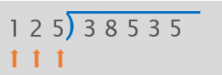### 3. We compare the 3 digits in the dividend with the 3 digits in the divisor.

Since 385 is greater than 125 we can start dividing.

### 4. We divide the first digits of the dividend and the divisor.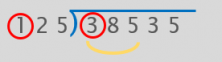3 divided by 1 is 3. We need to multiply 125 by 3 and see if it goes into 385.

125 x 3= 375.

We get 375 so we know it fits. We put the 3 in the quotient.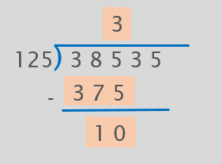### 5. We bring down the next digit of the dividend.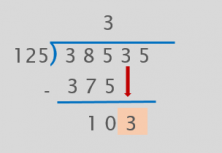We’ve brought down the 3 but now 125 doesn’t go into 103. So, how can we continue?

When this happens, we have to add a 0 to the quotient and bring down the next number in the dividend.

Now we can keep dividing.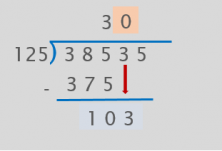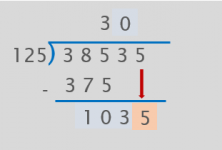First we divide 12 by 1 to see what number to put in the quotient. 12 divided by 1 is 12, and since it is greater than 10, we keep 9, the greatest one-digit number.

125 x 9 = 1125.

And 1125 doesn’t go into 1035.

Let’s try it with the next smallest number, 8.

125 x 8 = 1000.

That’s it!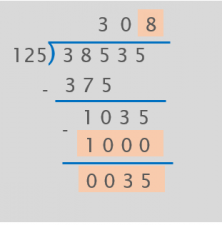We’ve finished the division.

38,535 divided by 125 gives us a quotient of 308 with a remainder of 35.

The only thing left to do, as always, is to check our work:

divisor x quotient + remainder = dividend

125 x 308+ 35 = 38,535

It works!

Now we know how to divide by 3 digits. If you want to keep learning more primary mathematics, register with Smartick and try it for free.